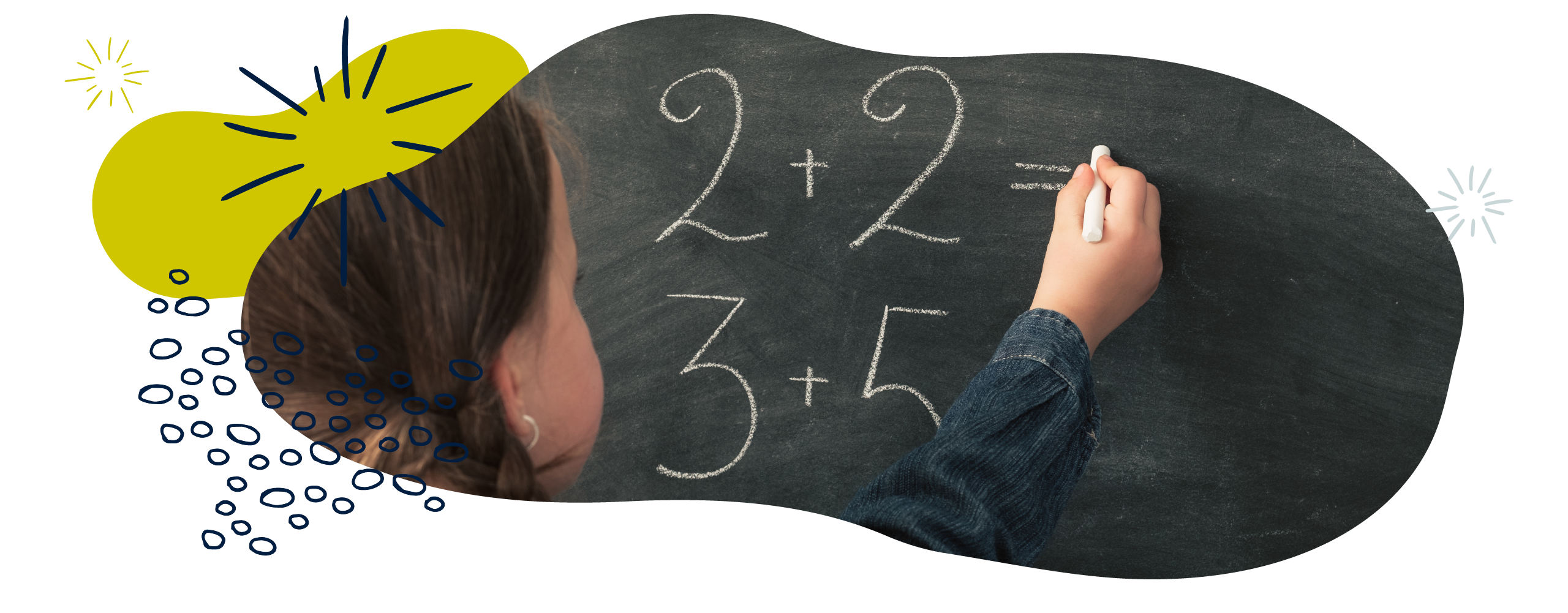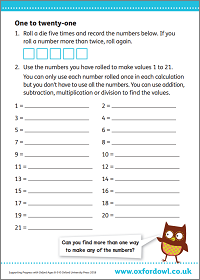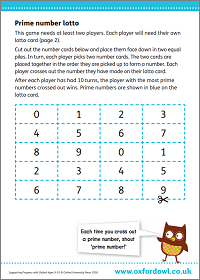We use cookies to enhance your experience on our website. By continuing to use our website, you are agreeing to our use of cookies. You can change your cookie settings at any time. Find out more# Calculation in Year 6 (age 10–11)

In Year 6, your child will often combine addition, subtraction, multiplication, and division in calculations. They will be able to apply all these operations to large numbers using a variety of written methods and some mental maths. They will learn about and use common factors, common multiples, and prime numbers.

The key words for this section are common factorfactor, and factor pair.

## What your child will learn

Take a look at the National Curriculum expectations for calculation in Year 6 (ages 10–11):

#### Multiply numbers up to four digits by numbers up to two digits

Your child should be able to use a variety of methods to multiply whole numbers up to four digits by whole numbers up to two digits, such as 2372 × 25. Methods could include:

• The area/grid method.
• Partitioning – for example, (2372 × 20) + (2372 × 5).
• Number facts – for example, 25 is a quarter of 100 and 2372 × 100 = 237,200. This means that 2372 × 25 is the same as 237,200 ÷ 4, which equals 59,300.
• Formal written methods such as long or short multiplication.

Your child will also be expected to keep practising their times tables so that they can solve problems quickly and accurately.

#### Divide numbers up to four digits by numbers up to two digits

Your child will practise lots of methods to solve division problems, including sharing and grouping using objects, chunking, and formal written methods of long and short division. They will be expected to divide whole numbers up to four digits by whole numbers up to two digits, for example, 2345 ÷ 23.

Your child will show their answers to division problems using remainders. A remainder is the amount left over after a number has been divided into another number (for example, 17 divides into 3 a total of 5 times, with a remainder of 2). Remainders can be represented as:

• Fractions – for example, 98 ÷ 4 = 24
• Decimals – for example, 98 ÷ 4 = 24.5
• By rounding – for example, 98 ÷ 4 = 24.5, which rounds up to 25.

Your child will be expected to interpret remainders depending on the context of the question. For example:

‘Buses can carry 30 people. There are 113 students going on the trip. How many buses will they need?’

This question is essentially asking 113 ÷ 30 = 3.77. But because the students can’t take 0.77 of a bus to school, it is appropriate to round up to 4. The students will need 4 buses.

#### Perform mental calculations, including with mixed operations and large numbers

Your child will be able to add, subtract, multiply, and divide large numbers in their head. They will be able to explain how they have solved a problem using objects, drawings, mathematical symbols, and words.

#### Identify common factors, common multiples, and prime numbers

Your child will be expected to recognise common factors, common multiples, and prime numbers and use this knowledge to solve problems. For example, they could use their understanding of common factors to find equivalent fractions:

They could work out that  =  because 3 is a factor of both 12 and 15.

#### Solve problems involving addition, subtraction, multiplication, and division

Your child will be expected to solve problems involving adding, taking away, multiplying, and dividing, often within the same calculation. They should know about different types of number (factors, multiples, primes, negative numbers, and so on) and be able to use this knowledge to help them solve problems.

Your child should also know about the order of operations (sometimes called BODMAS) and be able to use it in sums that involve adding, subtracting, multiplying and dividing.

#### Understand and use the order of operations

The order of operations (sometimes called BODMAS), refers to the order operations within a calculation should be completed to get the right answer. Watch this video for an explanation:

Your child should be able to solve calculations using the order of operations, including calculations that use brackets. They should know how brackets change what the calculation means. For example:

2 + 1 × 3 = 5
(2 + 1) × 3 = 9

#### Use rounding to check answers and make estimates

Your child will use rounding to estimate answers. They will then need to check that their answers are sensible and accurate.

They should be able to round answers to a specified degree of accuracy, for example, to the nearest 10, 20, 50, and so on.

Your child will be able to make judgments about what level of accuracy to round to in the context of a problem. For example, if adding 1,046,462 and 6,852,468, it might make sense to round to the nearest 1000, but when adding £3.15 and £2.74, it might make sense to round to the nearest pound or 10p.

#### Solve multi-step problems in contexts

In Year 6, your child will be expected to solve multi-step word problems. These are word-based maths problems that require your child to solve multiple calculations before coming to the answer.

The multi-step word problem could involve any combination of up to three operations (addition, subtraction, multiplication, and division). Your child should have a range of methods to solve these problems.

Your child will need to be able to choose an efficient method (or methods) to solve any given multi-step problem, and will need to be able to check their answer using a different method. They should also be able to explain how they have solved a problem and why they have chosen a particular method (or methods).

## How to help at home

### 1. Play the 1 to 21 game

You can help your child practise all four operations at home by playing the 1 to 21 game.

Ask them to roll a dice five times and record each number shown on the dice on a piece of paper. For example, 1, 4, 3, 5, 3. They then need to find a way to reach an answer of 1 using any operations (addition, subtraction, multiplication, and/or division) on the numbers.

Your child can only use each number once and they have to use at least two numbers in each calculation. For example, we could get the answer of 1 by calculating 3 ÷ 3, 5 – 4, 4 – 3, and so on. Then ask your child to find a calculation with the answer of 2 using any operation, then an answer of 3 and so on until they reach 21.

You could make this game even more challenging by saying that they have to use at least three, four, or even all five numbers to make it really tricky! Print out our activity sheet to have a go:

### Activity: One to twenty-oneFind ways to make all the numbers 1 to 21.

### 2. Play Four 4s

Play the game Four 4s to practise multiplying, dividing, adding, and taking away.

The aim is to make all the numbers from 1 to 20 using just the number 4 four times. Use a combination of operations (multiplying, dividing, adding, and taking away) to make calculations where the answers are the numbers 1 to 20.

You must use all four 4s, but you can put two together to make 44 as well as using operations.

1 = (4 ÷ 4) ÷ (4 ÷ 4)

2 = (4 × 4) ÷ (4 + 4)

… and so on.

### 3. Try lots of different methods

Encourage your child to use their knowledge of different calculation strategies to solve problems.

For example, when solving word problems, ask your child what exactly the problem is asking them to do. Which operations will they need? Does the problem involve equal sharing, or multiplication as scaling? Sometimes it can be useful to transform word problems into mathematical symbols to make it very clear what you need to do.

Encourage your child to estimate their answer by rounding before they solve questions with a formal method. This will help them check that their answer makes sense when they come to work it out properly.

Once your child has completed a question using their preferred method, see how many different ways they can find to solve the problem. They might find it useful to explain these methods to you with drawings or symbols. Talk about which method seems the most efficient or easiest for this particular problem. You may want to challenge them to make up their own similar problem to practise some more.

### 4. Explore factors, multiples, primes, and composite numbers

You can play a factor and multiple game with your child. Ask your child to choose an even number on a 100 square and cross it out. You then cross out a number that is either a factor or a multiple of that number. For example:

Start at 20.

A factor of 20 is 4.

A multiple of 4 is 40.

A multiple of 40 is 80.

… and so on. The game gets harder and harder as it goes on and more numbers are crossed out!

Continue to take it in turns to choose a number that is a multiple or a factor of the number that has just been crossed out by the other player. When a player cannot cross out any numbers, that player loses the game.

Your child will also need to explain why a number is prime or composite. Prime numbers are numbers that only have factors of 1 and itself. They can only be divided by 1 or themselves to get a whole number. 2, 3, 5, 7, 11, and 13 are all prime numbers. Composite numbers are numbers with more than two factors. 4, 6, 8, 9, 10, and 12 are all composite numbers.

Have more prime numbers or more composite numbers been crossed out in your game? Talk to your child about it. If you want to play another prime number game (or print out a number square), download the activity below:

### Activity: Prime number lotto### 5. Explore square and cube numbers

Your child will need to use and understand square and cube numbers.

A square number is created by multiplying an integer (whole number) by itself. For example:

9 is a square number because it can be made by multiplying 3 by 3.

25 is a square number because it can be made by multiplying 5 by 5.

144 is a square number because it can be made by multiplying 12 by 12.

A square number can also be represented in the shape of a square. A square with 3cm by 3cm sides has an area of 9cm². Ask your child to find some objects that are all the same size, and arrange them into a square. Notice how this makes a square number.

Cube numbers are formed by multiplying an integer (whole number) by itself three times. For example:

8 is a cube number because it can be made by multiplying 2 by 2 by 2.

27 is a cube number because it can be made by multiplying 3 by 3 by 3.

125 is a cube number because it can be made by multiplying 5 by 5 by 5.

A cube number can also be represented in the shape of a cube. You could ask your child to make a cube with building blocks or Lego. If they use blocks with sides of equal length (for example, 1cm by 1cm by 1cm), then any bigger cube they build will need to use a cube number of those blocks.